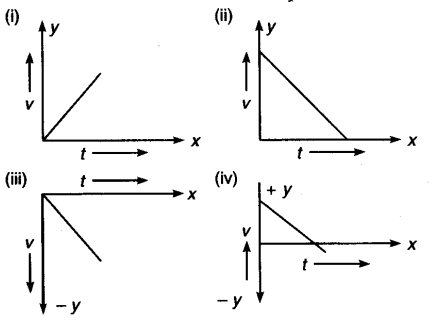# Give velocity-time graphs for motions having constant acceleration:

Give velocity-time graphs for motions having constant acceleration:
(i) When motion and acceleration are in positive direction,
(ii) When motion is in positive direction but acceleration is negative,
(iii) When motion is in negative direction and acceleration is also negative,
(iv) When motion of body is in positive direction and after some time in negative direction but acceleration is negative.